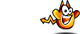Unit Conversion Software Web Widgets Loan Calculator Currency Rates Country Flags Unit Converter
 Faq Help# Pound-Force ConversionContact

## Pound-Force, unit of measure

To perform conversions between pound-force and other Force units please try our Force Unit Converter

Convert pound-force to:
newton, exanewton, petanewton, teranewton, giganewton, meganewton, kilonewton, hectonewton, dekanewton, decinewton, centinewton, millinewton, micronewton, nanonewton, piconewton, femtonewton, attonewton, dyne, joule/meter, joule/centimeter, gram-force, kilogram-force, ton-force (short), ton-force (long), ton-force (metric), kip-force, kilopound-force, ounce-force, poundal, pound foot/square second, pond, kilopondDownload Force Unit Converter
our powerful software utility that helps you make easy conversion between more than 2,100 various units of measure in more than 70 categories. Discover a universal assistant for all of your unit conversion needs - download the free demo version right away!Copyright © 1998-2009 UnitConversion.org Privacy & Terms | About | Faq | Help | Contact | Link to Us